APP## 做推荐业务，这4种机器效果测评方法你应该知道## R\P\A\F值

（1）二分类：每一个评估对象有唯一的标签，YES or NO。如低俗、标题党文章。

（2）多分类（机器需要识别的标签数包含3个及3个以上，一般情况下，每一种标签的识别结果都是我们的关注目标）

• 单标签，每一个评估对象有唯一的标签，选择大于等于3，如文章分类。
• 多标签，每一个评估对象有多个标签，如文章兴趣点、文章关键词。

### 1. 二分类

1. TP：预测正确，预测情况是P，因此真实情况也是P。
2. FP：预测错误，预测情况是P，因此实际情况是N。
3. FN：预测错误，预测情况是N，因此实际情况是P。
4. TN：预测正确，预测情况是N，因此实际情况也是N。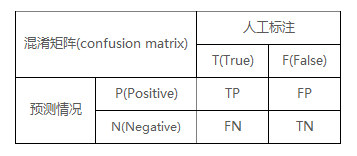（混淆矩阵示意图）（以上数据仅做理论说明，不做实际参考）• 召回率(R)=140/(140+11)=92.72%
• 精准率(P)=140/(140+40)=77.78%
• 准确率(A)=（140+4809）/(140+4809+40+11)=98.98%• 召回率(R)=140/151=92.72%
• 精准率(P)=140/180=77.78%
• 准确率(A)=（5000-40-11）/5000=98.98%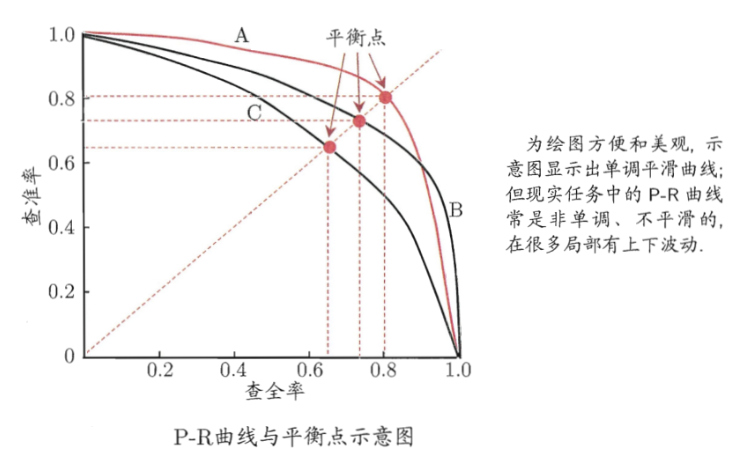（召回率也叫查全率，精确率也叫查准率）

F1 Score：召回率和精确率同等重要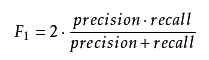• F2：召回率的重要程度是准确率的2倍
• F0.5：召回率的重要程度是准确率的一半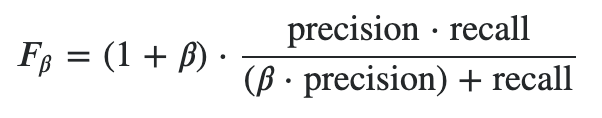（β大于0）

### 2. 多分类单标签

M_i : 表示机器识别是 i 类别，同时是正确的样本数

C_i : 表示机器识别是 i 类别的总样本数

N_i : 表示 i 类别的实际总数（即人工标记为是 i 类别的样本数）

D ：文章总数

K： 类别总数

• 精确率(A)=(M_0+M_1+……+M_K)/(C_1+C_2 + …… + C_K)
• 召回率(R)=(M_0+M_1+……+M_K)/(N_1+N_2+……+N_K)=(M_0+M_1+……+M_K)/D
• 覆盖率(Coverage)= 所有精确度符合要求的机器预测样本数/D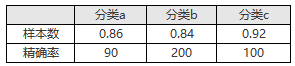### 3. 多分类多标签

M_i : 表示 i 标签识别正确的总样本数；

C_i : 表示 i 标签出现的总样本数；

N_i : 表示 i 标签实际总样本数（即人工标记为是 i 标签的总样本数）

K：表示标签集合的大小（即不同标签的个数）

• 准确率(A)=(M_0+M_1+……+M_K)/(C_0+C_1+……+C_K)
• 召回率(R)=(M_0+M_1+……+M_K)/(N_1+N_2+……+N_K)

## ROC、AUC

• 真正率(TPR)=TP/(TP+FN)，在“真”样本里预测正确的样本；
• 假正率(FPR)=FP/(FP+TN)，在“假”样本里预测错误的样本。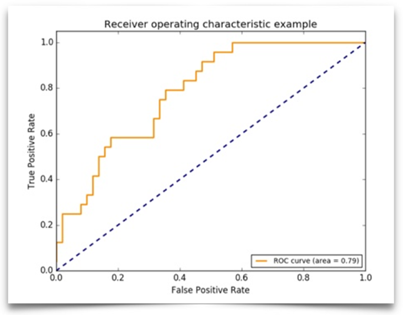## MAP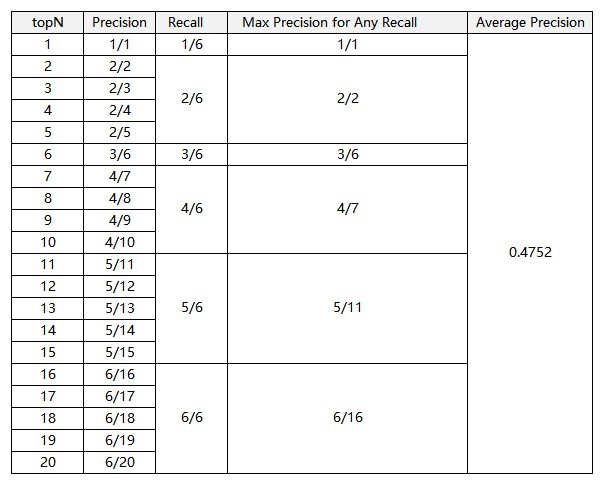AP衡量的是学出来的模型在给定类别上的好坏，而MAP衡量的是学出的模型在所有类别上的好坏，得到AP后MAP的计算就变得很简单了，就是取所有AP的平均值。

## CG/DCG/NDCG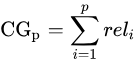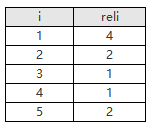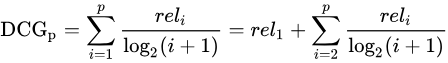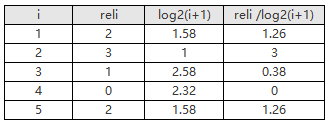DCG=1.26+3+0.38+0+1.26=5.9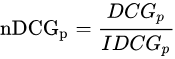IDCG为理想情况下（相关度降序排列）最大的DCG值：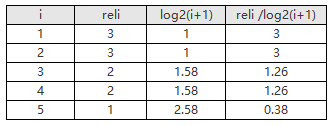### 参考文献：

• Willy_G《搜索：由流程框架到实现方法》，http://www.woshipm.com/pd/2866942.html
• 胖喵~《搜索评价指标——NDCG》，https://www.cnblogs.com/by-dream/p/9403984.html
• 残阳崔雪《性能指标（模型评估）之mAP》，https://blog.csdn.net/u014203453/article/details/77598997

3人打赏